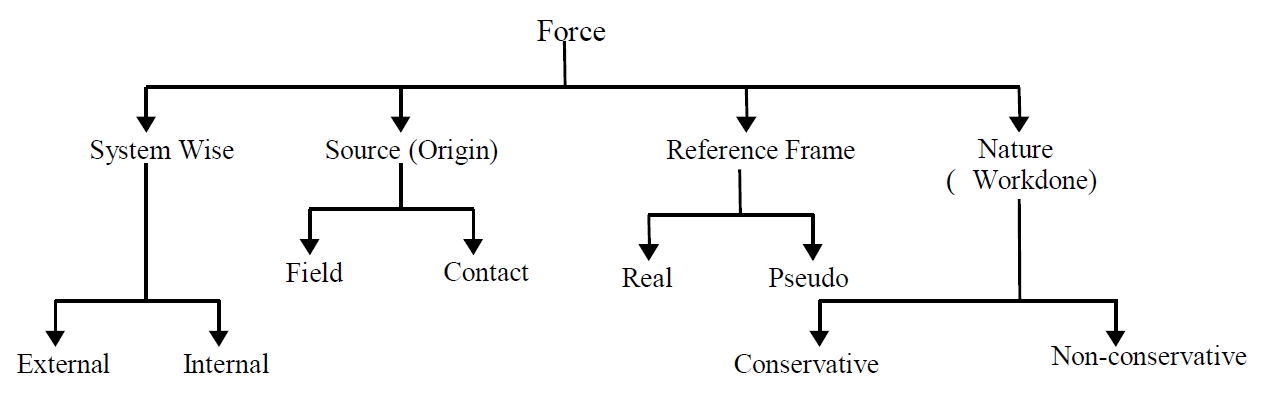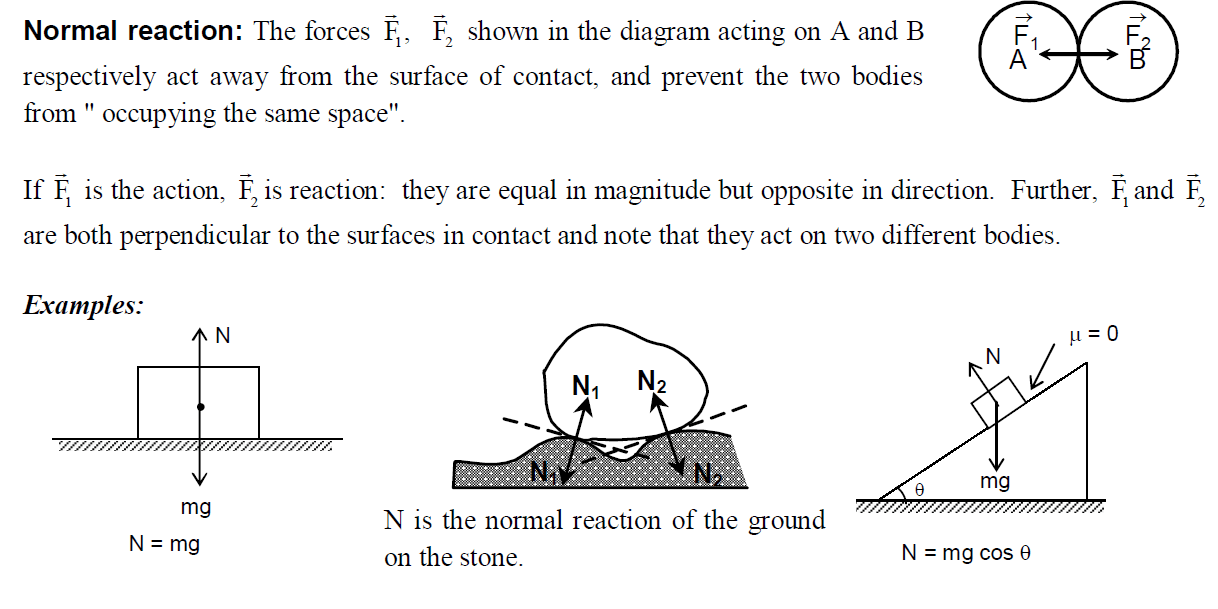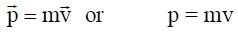# Laws of Motion (Study Notes)

## Topics to be covered

Force and inertia, Newton’s first law of motion; Momentum, Newton’s second law of motion, Impulse; Newton’s third law of motion; Law of conservation of linear momentum and its applications; Equilibrium of concurrent forces; Static and kinetic friction, laws of friction, rolling friction, lubrication; Examples of variable mass situation. Dynamics of uniform circular motion; Centripetal force, examples of circular motion (vehicle on level circular road, vehicle on banked road); Inertial and non- inertial frame (elementary idea).

## Introduction:

In the introduction of the preceding chapter “Kinematics” we studied that mechanics can be broadly classified into two categories namely “Kinematics and Dynamics”.

In kinematics our prime concern was to define the physical quantities like position, velocity, acceleration and to establish the relations among them. But we never tried to answer the questions like “What causes the bodies to move from one place to another? What makes the body to gain or loose its speed? . . . etc.

Dynamics is that branch of mechanics which gives not only qualitative but quantitative description of the above quantities. This branch of mechanics also explains few basic laws which governs the motion of bodies.

Before you start studying this chapter try to analyse the answers for the following questions:

(i) Why to set a ball into motion in playground someone has to kick it or throw it?
(ii) Why a navigator has to row the boat in still water to move the boat ?
(iii) Why the branches of a tree swing when wind blows ?

In all these cases one can observe that some external agency (like hand or legs of player or wind) is coming into contact with the respective objects that are going into motion.

(iv) When a ball is released from the top of a building, the ball falls by itself, though no one is pushing it in downward direction. Why ?
(v) When a piece of iron is placed near a magnet, magnet attracts it from certain distance itself. How ?

In these cases we can observe that even though no external agency is physically coming into contact with the objects still the objects are moving.

Hence we can conclude that an animate or inanimate external agency is required to change the state of a body (i.e. from rest to motion or vice versa). To understand the logic behind this type of questions more clearly, we should know about two basic physical quantities namely inertia and force.

Inertia: It is a very common observation for all of us that any book kept on our study table will not move by itself, i.e. until and unless it is acted upon by any external force, it will not change its state of rest.

To explain the reason behind this type of questions Italian scientist Galileo has defined a new physical quantity known as inertia. Though it was introduced by Galileo the effective use of this term and its usage for explaining the motion of the bodies was done by another reputed physicist, Sir Isaac Newton. “Inertia is an inherent property of a body by virtue of which it cannot change its state (i.e. rest or motion) by itself.”

It says that every body in the universe does have a property which is hidden in itself and because of this property the body is unable to change its state by itself, i.e. from state of rest to state of motion or vice versa or even it’s direction. This inertia is of 3 types, namely   (a) inertia of rest (b) inertia of motion and (c) inertia of direction

Inertia of rest: Inertia of rest is the inability of a body by virtue of which it can’t change its state of rest to state of motion. That means any body which is at rest continues to be in the state of rest only and it can’t go further into state of motion by itself.

Examples:

(i) Passengers standing or sitting loosely in a bus experience jerk in the backward direction when the bus suddenly starts moving. This is due to the fact that when the bus suddenly starts its motion, the lower parts of the human body shares the motion but the upper part tends to remain at rest due to inertia of rest.

(ii) When a bullet is fired into a tightly- fitted glass pane from a reasonably close range, it makes a clear circular hole in the glass pane. This is due to the fact that particles of glass around the hole tend to remain at rest due to inertia of rest. So they are unable to share the fast motion of the bullet.

Inertia of motion: Inertia of motion is the inability of a body by virtue of which it can not change its state of uniform motion along a straight line to state of rest. That means any body which is in uniform motion can’t come to rest by itself until and unless some external force acts on it.

Examples:

(i) A passenger standing in a moving bus falls forward when the bus stops suddenly. This is due to fact that the lower part of the body comes to rest along with the bus but the upper part of the body remains in a state of motion on account of “inertia of motion”.

(ii) An athlete runs for some distance before taking a long jump. In this way, the athlete gains momentum and this inertia of motion helps him in taking longer jump.

Inertia of direction: Inertia of direction is inability of a body by virtue of which it can’t change its direction by itself. This means a body moving along a straight line can’t change its direction by itself, until and unless it is acted upon by any external force.

Examples:

(i) When a running car suddenly takes a turn, the passengers experience a jerk in the outward direction. This is because the passengers tend to maintain their original direction of motion due to inertia of direction.

(ii) A stone tied to one end of a string is whirled in a horizontal circle. When string breaks, the stone tends to fly off tangentially along a straight line. This is due to inertia of direction.

Note: The mass of the body is the indirect measure of the inertia of that body.

FORCE

“Force is that which pushes or pulls the body or tends to change the state of rest or of uniform motion in a straight line.”

(a) It produces or tries to produce motion in a body at rest.
(b) It stops or tries to stop a moving body.
(c) It changes or tries to change the direction of motion of body.
(d) It produces a change in the shape of the body.

### CLASSIFICATION OF FORCES

There are different types of forces in our universe. Based on the nature of the interaction between two bodies, forces may be broadly classified as under(a) Contact force: The force exerted by one surface over the surface of another body when they are physically in contact with each other is known as contact force.

If two surfaces that are coming into contact are perfectly smooth, then the entire contact force will act only perpendicularly (normal) to their surface of contact and it is known as “Normal force or Normal reaction.”

If two surfaces that are coming into contact are rough surfaces, then one component of this contact force acts perpendicular to their surface of contact and the other component of this force acts in tangential direction to their surface of contact and this component is known as “force of friction.”

Normal Reaction, Tension, Friction, etc. are the examples of various contact forces.Friction: It is a force that acts between bodies in contact with each other along the surface of contact and it opposes relative motion between the two bodies. The direction of frictional force on A is opposite to that of direction of frictional force on surface B and magnitude is same for both.Tension (T): When a string, thread or wire is held taut, the ends of the string or thread (or wire) pull on whatever bodies are attached to them in the direction of the string. This force is known as Tension. If the string is massless then the tension T has the same magnitude at all points throughout the string.

Examples:
(i) Tension in a string: For a block A pulled by a string,

(ii) The direction of tension is always away from the point of attachment to the body. In the given figure two segments of tension act at O towards A and B. For the wedge, there are two segments of thread at the point of attachment O to the body. Hence, two tensions act on the wedge; one along OB and the other along OA.

Hence a tension acts away from the point of attachment along BO.

Spring forces: Whenever a spring is compressed or extended, the elastic force developed in the spring which helps the spring to restore its original position is known as spring force.
In an extended (or compressed) spring, force is proportional to the magnitude of extension (or compression).F α x, in magnitude, but opposite in direction. So, F = – kx, where ‘k’ is a positive constant, also known as the spring constant of the spring. x is the compression or elongation from the natural length.

Example:
In case of the spring the tensions are oppositely directed on the block ‘m’ and on the roof R.

(b) Non-contact force: Bodies can exert forces on each other without actual physical contact. This is known as action at a distance. Such forces are known as non-contact forces (or) field forces, e.g. gravitational force, electrostatic forces, etc.

For the moment, we deal with actual forces. Suffice it to say that there exist pseudo-forces acting in a non-inertial frame of reference, which we will discuss later.

Forces may be conservative or non-conservative depending on whether work done against them by an external agent is recoverable or otherwise. This will be discussed in later chapters.Now having understood about inertia and force, we can easily understand the three basic laws of motion which are known as Newton’s laws of motion.

## Newton’s first law

#### Every body continues to be in the state of rest or of uniform motion in a straight line until and unless it is compelled to change the state of the body by an unbalanced force.

For better understanding we can divide this statement into two parts.

(i) “Every body continues to be in its state of rest until and unless some external force compels it to change the state of rest.”
This part of the law is self explanatory and self evident as we come across several examples in our daily life like all inanimate objects will continue to be in the same place where they are put until they are disturbed by some external agents.

(ii) “Every body continues in its state of uniform motion in a straight line unless external force compels it to change that state.”

The second part of the statement can’t be readily understood as on the surface of the earth because of various types of frictional or resisting forces. For example when a ball is rolled on a horizontal surface the ball will come to halt after some time however smooth the surface may be, as we can’t eliminate force of friction completely.

Momentum (Linear):
Till now we studied about inertia (translational) which is the inability of a body. Now we will study about another physical quantity called ‘momentum’ which is the ability of body.

Momentum is defined as the ability of a body by virtue of which it imparts or tends to impart its motion along a straight line.

Mathematically, momentum (p) is measured as the product of mass (m) and velocity (v) of the body. As velocity is a vector quantity, momentum is also a vector quantity.Exercise 3.

(i) If a body is at rest, can we say that no force is acting on the body?

(ii) A car and a lorry are travelling with same velocity on a straight horizontal road. Which of the two has got greater momentum?Newton’s second law of motion

We have already studied the Newton’s 1st law which has given us a qualitative idea about force. Now, we will study about Newton’s 2nd law which gives us a quantitative idea about force.

Whenever a cricketer catches a ball he allows a longer time for his hands to stop the ball. Otherwise the ball will hurt the cricketer. If you observe this incident carefully you can easily understand that cricketer is applying some force on ball in order to make the momentum of the body zero. And also we can understand that the magnitude of the retarding force that cricketer applies on the ball in order to stop depends on two
factors.
(1) The momentum of the ball and
(2) Time for which he is applying the force
These type of observations lead Newton to state his second law of motion.

The rate of change of momentum of a body is directly proportional to the applied force and the change takes place in the direction of the force.Note (1): The second law of motion is a vector law. These are actually three equations, one for each component of the vectors:Note (2): The second law of motion is strictly applicable to a single point particle. The force F here stands for net external force on the particle.

### Exercise 4. A body is acted upon by a number of external forces. Can it remain at rest ?

Announcements

Join our Online JEE Test Series for 499/- Only (Web + App) for 1 Year

Join our Online NEET Test Series for 499/- Only for 1 Year